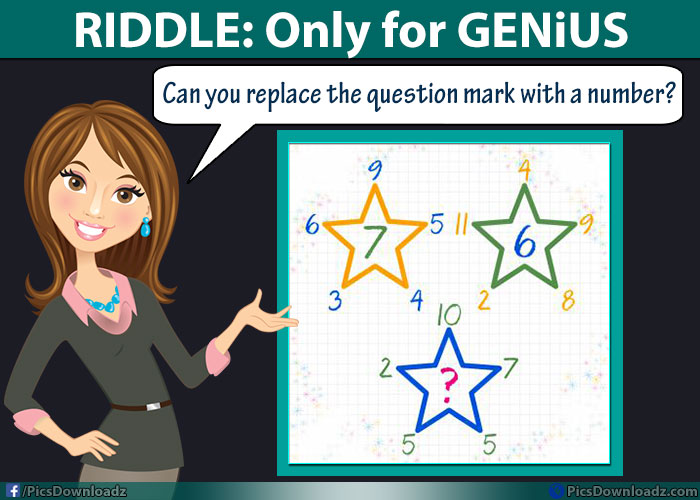This post may contain affiliate links. This means if you click on the link and purchase the item, We will receive an affiliate commission at no extra cost to you. See Our Affiliate Policy for more info.

10, 7, 5, 5, 2 = ? Math Riddle Only for Genius – Replace Question Mark Puzzles

0
1627

Replace the question mark with a number puzzle! Math Brainteasers Riddles – Only for Genius with the answer!

Hello and welcome back! Here we come’s with an interesting, confusing and difficult math puzzle. In this genius brainteaser puzzle, you will have to find out the number which replaces the question mark.

There are three stars, each star has some numbers. You have to find the logic behind these number and how they relate to the middle one. After that apply the formula on the third star and get the answer.

This puzzle will blow your mind, take the challenge and solve the puzzle. Check the puzzle image below:

Genius Math Puzzle ImageFirst Star:

The logic behind this puzzle is in following ways:

9+5+4+3+6 = 27, now 2×7 = 14

now if the number is in two digits then multiply both with each other; 2×7 = 14

Now divide the number with 2 i.e  14 ÷ 2 = 7

Second Star:

Same logic; 4+9+8+2+11 = 34

3×4 = 12, Now 12 ÷ 2 = 6

Third Star:

10+7+5+5+2 = 29

2 × 9 = 18, Now 18 ÷ 2 = 9 Ans.

I now it sucks! But that’s why it’s called Genius Puzzle!  😎

So, how’s the puzzle? Hope you found this puzzle interesting and challenging. Share this puzzle with your friends. Connect with us for more interesting and funny stories updates.

For now, Enjoy reading and take care!

Search items:

Math Puzzles Images, Math Puzzles Only for Genius, Math Puzzles with Answer, Genius Math Riddles, Difficult Math Puzzles, Best Brainteasers Puzzles, Hard Math Puzzles Images, Puzzles for Facebook, Whatsapp Puzzles Images Download, Puzzles for Whatsapp, Exams Puzzles Questions,